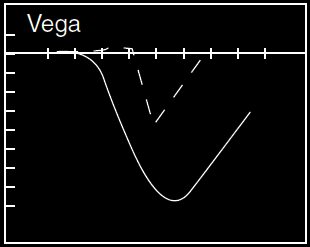Call Ratio Spread Options Greeks: Delta, Gamma, Rho, Vega, Theta: Options, Futures, Derivatives & Commodity Trading

# Call Ratio Spread Options Greeks: Delta, Gamma, Rho, Vega, Theta

Details about Ratio Call Spread Options Greeks: Delta, Gamma, Rho, Vega, Theta
Continuing further from our previous article Ratio Call Spread Options Trading: Profit & Loss Calculations, here are the Details about Greeks (Delta, Gamma, Rho, Vega Theta) for Ratio Call Spread Option Trade
Dotted line indicates a shorter maturity option Greeks while the solid line represents the long maturity option Greeks.

## Short Call Butterfly Option Greeks: Delta, Gamma, Rho, Vega, Theta

Delta for Ratio Call Spread Option:The delta value becomes more and more insensitive as the underlying stock price falls lower and lower and hence on the lower side, the delta value is zero.
On the higher side, it increases in magnitude but remains negative indicating the negative effect on the option trader position with the increasing underlying stock prices.Gamma is the first derivative of delta and second derivative of the underlying stock price. Hence, it has a high value around the higher strike price which is also the turning point and then it tapers downwardsTime decay derivative - good for option trader in Ratio Call Spread and maximum benefit comes when the underlying stock prices don’t move much. As they break away into the loss making regions, the theta value diminishes indicating insensitivity to time decay in loss making regions.Increasing volatility is bad for the Ratio Call Spread position as that increases the possibility of underlying stock price breaking into the loss making regions either on the upside or the downside.

Effect of interest rate changes on the option position is measured by Rho. When the underlying stock prices are on the rise, the increase in interest rates is bad for the Ratio Call Spread.Posted by IT Correspondent See All Articles with